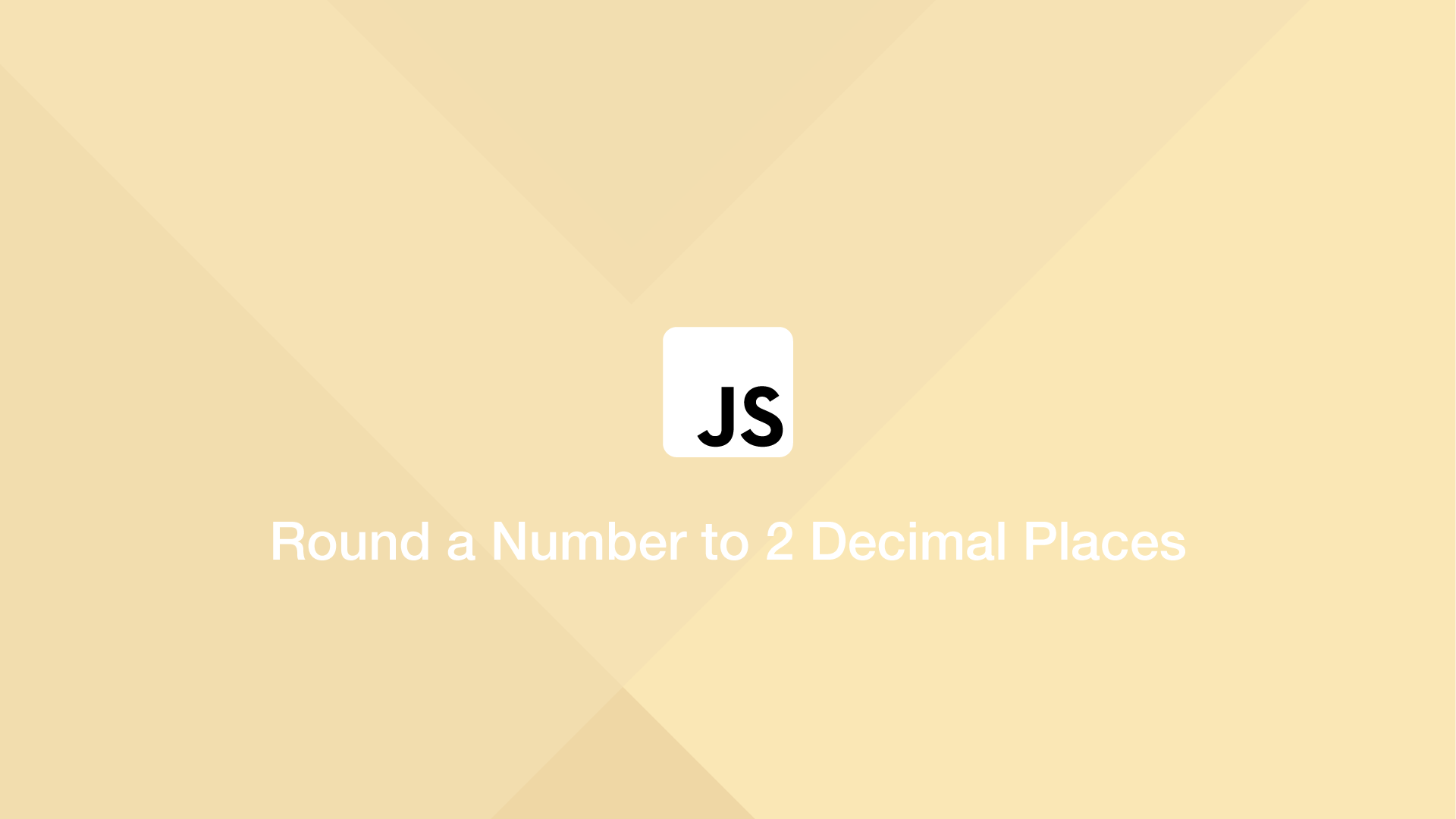# Round a Number to 2 Decimal Places in JavaScriptTo round a number to decimal places in Javascript, use either the .toFixed() or Math.round() function. In this tutorial we will learn how to use both methods.

## Rounding a Number to 2 Decimal Places with .toFixed()

The .toFixed() function will round a number to a fixed number of decimal places. Pass the number to round before the function and the number of decimal places to round to as the first argument.

``````var num = 22.22434;
var num2 = 1.004

var res = num.toFixed(2);
console.log(res);
``````
``````22.22
``````

Note – This method will not work on numbers like 1.004.

## Rounding a Number in JavaScript to 2 Decimal Places with Math.round()

To round numbers to 2 decimal places with improved accuracy, use the Math.round() function with the Number.EPSILON utility like this:

``````var num = 22.22434;

var res = Math.round((num + Number.EPSILON) * 100) / 100;

console.log(res);
``````
``````22.22
``````

Note – This method will not work on numbers like 1.004.

## Round Numbers with Multiple 0's Decimal Places

The two previous methods will not round numbers like 1.005 to two decimal places accurately. To get around this, we will use the `.toPrecision()` function to remove rounding errors before getting the final result.

``````function round(num) {
var m = Number((Math.abs(num) * 100).toPrecision(15));
return Math.round(m) / 100 * Math.sign(num);
}

console.log(round(1.005));
``````
``````1.01
``````

#### Related Tutorials### How to Round Decimals Up and Down in JavaScript

September 08, 2020### How to Round Numbers Up and Down in Python

September 28, 2020### How to Convert a String to a Number in JavaScript

August 20, 2020### Truncate Float in Python

June 29, 2021### Round a Double to Two Decimal Places in Java

March 16, 2022### How to Generate Random Numbers in JavaScript

September 13, 2020